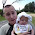Friday, February 22, 2013

Different attitudes to Mathematica

The cartoon is from Saturday Morning Breakfast Cereal.
I thank my son Luke for bringing it to my attention.

This does raise an important issue. To what extent should students be encouraged or allowed to use Mathematica and Matlab?
It seems to me there needs to be a balance: between learning to use a powerful tool an understanding how it works.

For example, I think it is very important that students learn to sketch graphs of simple functions. This provides intuitive understanding and a way of checking that the computer is giving a reasonable answer.
Perhaps it is no different from pocket calculators.

Here is Ben Powell's comment on this post. It took me a while to get it!

1.I would say that students should use Mathematica to do everything it can do. This is partly because I'm a believer in specificity: students will learn best when they are doing exactly what they are learning to do.

Having said that, there are two things to note.

Firstly, it's important to understand concepts before embarking on Mathematica use. My favourite example is "what does the dx in an integral really mean". Anyone who doesn't draw a picture, labelling dx, doesn't understand integral calculus yet (or any more?!).

Secondly, often understanding how different features of a question affect the features of the answer provide physical intuition. It's important to understand this, perhaps by actually doing the problem by hand. But there's no hand in letting Mathematica do it first.

I agree that it's important to recognise whether an answer is reasonable, but I'm far more likely to make mistakes by hand than using Mathematica.

2.hint about the powell joke?

i typed it in myself to see what was going on, and im clearly being wayyyy to literal, but i got an answer and it wasnt -1.

1.Hint.

It is tricky and I had to think a while.

1.
Note the integrand is a positive function and so
the integral must be a positive number.
Hence, clearly -1 is a nonsense answer.

2.
I think you need to change variables
x=t+u
y=t-u
and evaluate the Jacobian of the transformation matrix.
The double integral then factorises into the square
of \int^-\infty^+\infty dx sech^2(x) = 1
using fact derivative of tanh x is sech^2(x).
So I think the answer (in my head) is +1.

2.I always think that assuming that because the integrand is positive, the integral should be positive, but then I get a little skeptical because the sum of the positive integers is -1/12, and this can be used to derive the Casimir force, so I don't know what to believe.

Just being silly, before people start bagging me.

Incidentally, at the Windsor Low Dimensional Condensed Matter School last year, Daniel Loss gave a similar cautionary tale about Mathematica, how it had given him a wrong result for a specific calculation, and then he discovered that there are very many examples of wrong calculations it gives.

All in all it's pretty awesome though, I think.

3.I think you need to be running mathematic version 8 to get this result. Version 9 gives 2. So at least one of them is wrong. I also got +1 when I tried it analytically (using the transformation as Ross).

3.Computer algebra is a powerful tool, but it lacks something pretty important: creative problem solving ability.

It also seems that Mathematica can become less useful if constraints on the relevant sets are needed. By default, it gives all the answers it can "think" of, regardless if they make sense in the context of the intended limits of the sets involved. However, in order to get beyond this, one needs to start learning the programming language itself, which may defeat the time savings of using it in the first place.

A big problem with Mathematica is that, although it is very, very powerful, the documentation is NOT up to task with the power of the program. There's "The Mathematica Guidebook" series... but I casually estimate the length of the whole series at >10K pages.

There is an O'Reilly "cookbook" that is pretty good, for reference and introduction to certain key features... but I do get the impression that in order to master the program probably takes decades of practice.

1.Creative problem solving is important, however the dominant majority of problems can be solved using one or another technique that is already well-known--just not necessarily to the researcher facing the problem. Thus, on average, I would say that Mathematica saves a lot of time (and once you have the answer you should verify it manually). Also, my experience suggests that if you find yourself reading about some special function hitherto unknown to you that Mathematica output when solving your equation, consider verifying the equation once again... :-)

4.Using Mathematica can be really helpful. Check out this review of Mathematica. http://www.statisticsblog.com/2013/03/review-of-mathematica-9-and-r-link/